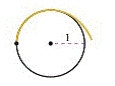Chapter 10.3, Problem 94E

Chapter
Section
Textbook Problem

# Involute of a Circle The figure shows a piece of string tied to a circle with a radius of one unit. The string is just long enough to each the opposite side of the circle. Find the area that is covered when the string is unwound counterclockwise.Figure for 94

To determine

To calculate: The area is covered when string is unwound counters clockwise as given in the figure below:Explanation

Given:

A piece of string tied to circle with radius of one unit and string is just long enough to reach the opposite side of circle.

The figure given is,

Formula used:

The area under the curve,

A=abydx=abydxdtdt

Calculation:

The string is unbounded by the angle of θ, therefore, find the locus of the string,

From the above figure, to find the co-ordinate of the point B.

The arc length AE is,

AE=rθ

The arc length AE is equal to the AB. Therefore,

AB=AE=rθ

The length of OC is,

OC=OAcosθ=rcosθ

The length of AC is,

AC=OAsinθ=rsinθ

Consider the triangle ΔOCA, then the angle OAC is,

COA+OAC+ACO=180°θ+OAC+90=180°OAC=90°θ

Since, the line AB is perpendicular to the line OA. Therefore,,

OAC+CAB=90°

Substitute OAC=90°θ,

OAC+CAB=90°90°θ+CAB=90°CAB=90°90°+θ=θ

The length of DB is,

DB=ABsinθ=rθsinθ

Now, the co-ordinate of point B is (x,y). So, to find the x-coordinate of the point B

### Still sussing out bartleby?

Check out a sample textbook solution.

See a sample solution

#### The Solution to Your Study Problems

Bartleby provides explanations to thousands of textbook problems written by our experts, many with advanced degrees!

Get Started

#### Find more solutions based on key concepts